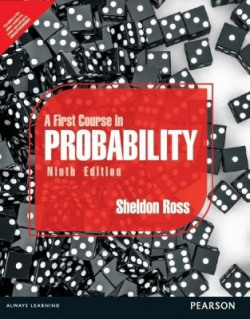EXPIRED

### Flipkart | Book LOOT

`A First Course in Probability is a comprehensive book for mathematics students and undergraduates studying statistics and probability. This book is aimed at an introductory course in probability and lays down the basic principles underlying a study of probability. It puts the subject in a practical context to help students prepare for a higher course in the subject. The book discusses Combinatorial Analysis, Random Variables, Expectation and Limit Theorems. In addition the book provides an ample amount of examples and applications to help students u`
759
95